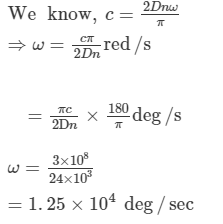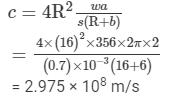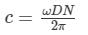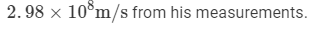Courses

# Speed Of Light JEE Notes | EduRev

## JEE : Speed Of Light JEE Notes | EduRev

The document Speed Of Light JEE Notes | EduRev is a part of the JEE Course HC Verma and Irodov Solutions.
All you need of JEE at this link: JEE

Page No.447

Ques 1:The speed of sound in air is 332 m s−1. Is it advisable to define the length 1 m as the distance travelled by sound in 1/332 s?

Ans: No, it is not advisable to define the length 1 m as the distance travelled by sound in 1/332 s because the speed of sound is affected by various factors such as temperature, humidity and nature of medium. So, it cannot be said that the distance travelled by sound in 1/332 s will be 1m, less than 1 m or more than 1m

Ques 2:Consider Galileo's method of measuring the speed of light using two lanterns. To get an accuracy of about 10%, the time taken by the experimenter in closing or opening the shutter should be about one tenth of the time taken by the light in going from one experimenter to the other. Assume that it takes 1/100 second for an experimenter to close or open the shutter. How far should the two experimenters be to get a 10% accuracy? What are the difficulties in having this separation?

Ans 2:We have speed of light = 299792458 m/s

To have a accuracy of 10% the light has to travel 1/10th of a second between the observers so,

Distance travelled by the light in 0.1 s = 0.1×299792458= 29979 km.

The difficulty in separation of that distance will be the curvature of earth. As the earth’s surface is curved, light from one of the experimenters won’t reach the other.

Ques 3:In Fizeau method of measuring the speed of light, the toothed wheel is placed in the focal plane of a converging lens. How would the experiment be affected if the wheel is slightly away from the focal plane?

Ans:If the wheel is placed away from the focal plane the light returning light rays will fall in an extended area of the wheel, this will let image to appear even when the light ray is blocked by one of the teeth of the wheel.

Page No.448

Question 1:In an experiment to measure the speed of light by Fizeau's apparatus, following data are used: Distance between the mirrors = 12.0 km,

Number of teeth in the wheel = 180.

Find the minimum angular speed of the wheel for which the image is not seen.

Ans 1:Distance between the mirrors (D) = 12.0 km = 12 × 103 m

Number of teeth in the wheel (n) = 180

Now we apply Fizeau's apparatus

Speed of light, c = 3 × 108 m/sHence, the required angular speed of the wheel for which the image is not seen is 1 . 25 × 10 4 deg / sec 1.25×104 deg/sec

Ques 2:In an experiment with Foucault's apparatus, the various distances used are as follows:

Distance between the rotating and the fixed mirror = 16 m

Distance between the lens and the rotating mirror = 6 m,

Distance between the source and the lens = 2 m.

When the mirror is rotated at a speed of 356 revolutions per second, the image shifts by 0.7 mm. Calculate the speed of light from these data.

Ans 2:

Distance between the rotating and the fixed mirror (R) = 16 m

Distance between the lens and the rotating mirror (b) = 6 m

Distance between the source and the lens (a) = 2 m

Mirror is rotated at a speed of 356 revolutions per second

⇒ ω = 356 rev/s= 356 × 2 π rad/sec

Shift in the image (s) = 0.7 m = 0.7 × 103 m

In Foucault experiment, speed of light is given byTherefore, the required speed of light is 2.975 × 108 m/s

Ques 3:In a Michelson experiment for measuring speed of light, the distance travelled by light between two reflections from the rotating mirror is 4.8 km. The rotating mirror has a shape of a regular octagon. At what minimum angular speed of the mirror (other than zero) the image is formed at the position where a nonrotating mirror forms it?

Ans:Distance travelled by light between two reflections from the rotating mirror (D) = 4.8 km = 4.8 × 103 m

Number of faces of the mirror, N = 8

Angular speed of the mirror, ω = ?

In Michelson experiment, the speed of light (c) is given bywhere,

ω = angular speed

N = number of faces in the polygon mirrorHence, the required angular speed is 7.8 × 103 rev/s.

Ques 4:In the original Fizeau method, the light travelled 8.6 km and then returned. What could be the difficulty if this distance is taken as 8.6 m?

Ans:There is no difficulty if the distance travelled by light is decreased. In this method, light has to travel a large distance of 8.6 km. So, the intensity of the light decreases considerably and the final image becomes dim. If somehow this distance is decreased, the final image is dark due to the increased light intensity.

Ques 5:What is the advantage of using a polygonal mirror with larger number of faces in Michelson method of measuring the speed of light?

Ans:The advantage of using a polygonal mirror with larger number of faces in the Michelson method is it gives the value that is very near to the accurate value and minimises the error.
Objective I

Ques 1:Light passes through a closed cylindrical tube containing a gas. If the gas is gradually pumped out, the speed of light inside the tube will

(a) increase

(b) decrease

(c) remain constant

(d) first increase and then decrease

Ans:(a) increase

If the gas is gradually pumped out, a vacuum will be created inside the closed cylindrical tube, and experimentally, light travels at the fastest speed in vacuum as compared to any other medium.

Ques 2:The speeds of red light and yellow light are exactly same

(a) in vacuum but not in air

(b) in air but not in vacuum

(c) in vacuum as well as in air

(d) neither in vacuum nor in air

Ans:(a) in vacuum but not in air

Different wavelengths travel at different speeds through different media.  In vacuum, the speeds of both the red light and yellow light are same but are different in air due to some optical density of air. Both wavelengths act in a different way in the air.

Ques 3:An illuminated object is placed on the principal axis of a converging lens so that a real image is formed on the other side of the lens. If the object is shifted a little,

(a) the image will be shifted simultaneously with the object

(b) the image will be shifted a little later than the object

(c) the image will be shifted a little earlier than the object

(d) the image will not shift

Ans:(b) the image will be shifted a little later than the object

Light rays emitting from a source have to cover some optical distance to form an image of the source on the other side of the lens. So, when a light source is shifted by some distance on the principal axis, then the light rays emitting from the new position of the source take some time to form a shifted image of the object on the other side of the lens. However, this delay is very small because the speed of light has a very larger value.

Objective II

Ques 1: The speed of light is 299, 792, 458 ms−1

(a) with respect to the earth

(b) with respect to the sun

(c) with respect to a train moving on the earth

(d) with respect to a spaceship going in outer space.

Ans:(a), (b), (c) and (d)

The speed of light is a fundamental constant, and with respect to any inertial frame, it is independent of the motion of the light source.

Ques 2:Which of the following methods can be used to measure the speed of light in laboratory?

(a) Roemer method

(b) Fizeau method

(c) Foucault method

(d) Michelson method

(c) Foucault method

Foucault gave the first laboratory method to find the velocity of light. He obtained a value ofQues 3:Which of the following methods can be used to measure the speed of light in water?

(a) Roemer method

(b) Fizeau method

(c) Foucault method

(d) Michelson method

Ans:(c) Foucault method

Foucault method can be used to measure the speed of light in water. One of the advantage of this method is that one can put some transparent medium (or water) between two mirrors to measure the speed of light in that medium (or water).  Foucault observed that the velocity of light in water is less than that in the air.

Offer running on EduRev: Apply code STAYHOME200 to get INR 200 off on our premium plan EduRev Infinity!

76 docs

,

,

,

,

,

,

,

,

,

,

,

,

,

,

,

,

,

,

,

,

,

;